# How to Sum the Smallest N Values in Excel

Sometimes we may want to sum the first smallest N numbers in a range in Excel. In this article, we will show you the method of “SUM the Smallest N Numbers” by a simple formula which consist of SUMPRODUCT and SMALL functions. SMALL can return smallest values based on criteria, SUMPRODUCT can sum up these smallest values.

Through a simple instance, we will introduce you the syntax, argument and the usage of SUMPRODUCT and SMALL functions. We will let you know how the formula works step by step clearly. After reading the article, you may know that in which situations we can choose SUMPRODUCT function to sum data.

## EXAMPLE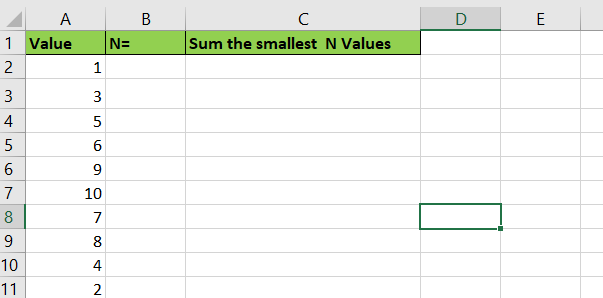In the ‘Value’ column, there are 10 values from ‘1’ to ‘10’ in improper order. Now we want to sum the smallest 4 values from this list, how can we get the correct result?

Actually, we can sort these numbers from smallest to largest firstly by ‘Sort A-Z’ in excel.

Click ‘Data’ tab, under ‘Sort & Filter’ section click ‘Sort Smallest to Largest’ icon.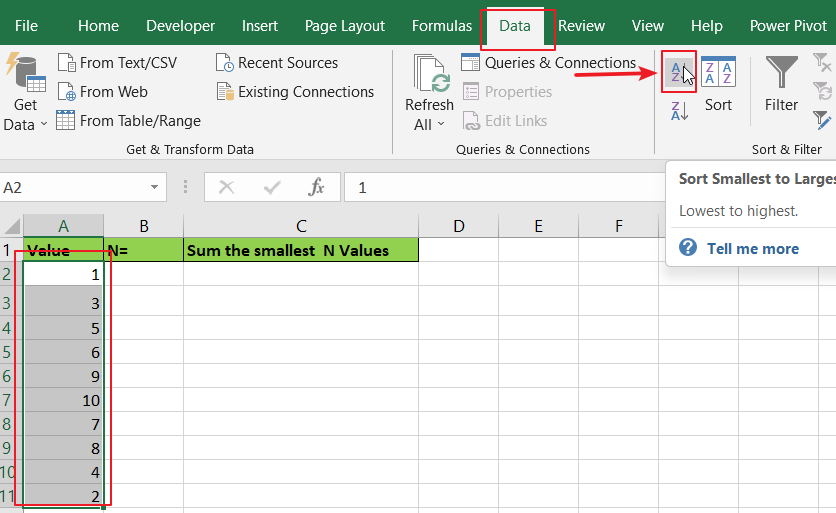Then you can find numbers are ordered properly.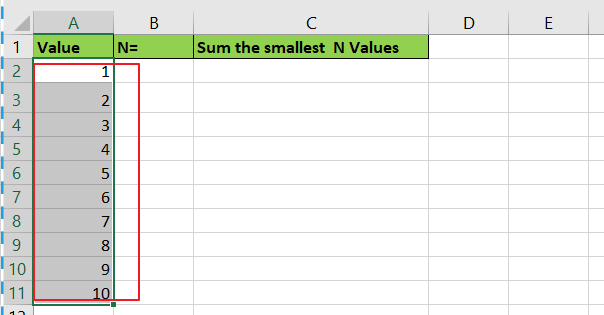Then you can enter SUM function in B2 and select the first four numbers ->cell reference A2:A5 in the list.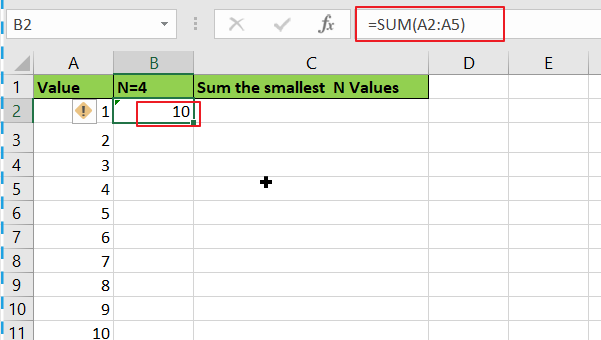Then you can get the sum of the smallest four values.In this way, we can get result correctly. But we can also get sum conveniently and correctly by just enter a formula. As we want to sum the smallest four numbers from range A2:A11, we can find the smallest N number or numbers by SMALL function actually, and then use SUMPRODUCT function to sum array directly.

## FORMULA – APPLY SUMPRODUCT FUNCTION

Step 1: In B2, enter the formula

`=SUMPRODUCT(SMALL(A2:A11,{1,2,3,4})).`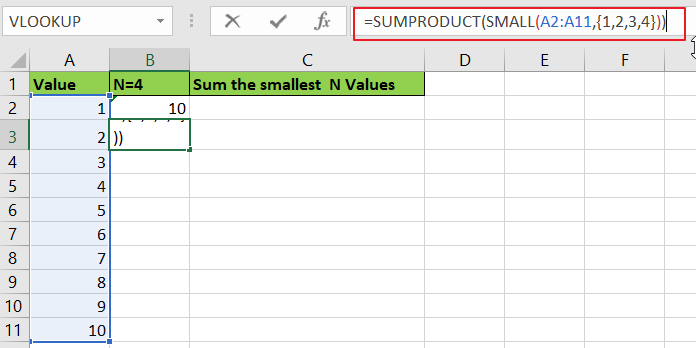Step 2: Press Enter after typing the formula.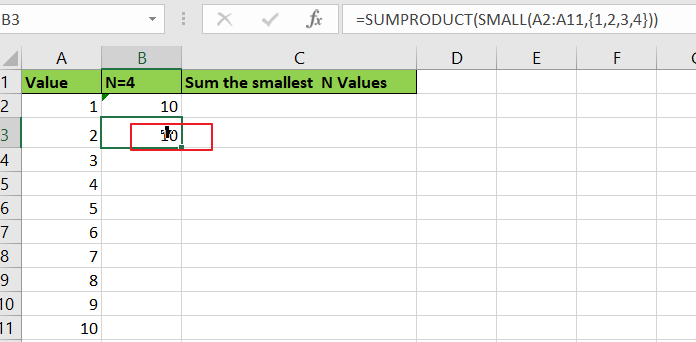We can see that this time we also get correct result 10. The formula works correctly.

### FUNCTION INTRODUCTION

SUMPRODUCT function can be seen as SUM+PRODUCT.

For SUMPRODUCT function, the syntax is:

`=SUMPRODUCT(array1,array2,array3, ...)`

The values in arrays are multiplied correspondingly, then sum up the products. For example, enter =SUMPRODUCT({1,2,3},{2,3,4}) in any cell, the we can get value 20, it equals to 1*2+2*3+3*4=20. If there is only array in the formula, SUMPRODUCT will sum the numbers in the array.

SUMPRODUCT function allows entering texts, logical operators and expressions like (product=cap), texts should be enclosed into ().

SMALL function can return the Nth smallest value in a range.

For SMALL function, the syntax is:

`=SMALL(array,k)`

If k is a number, it returns the smallest number in the range; if k is an array like {1,2,3}, it returns the smallest three numbers in the range. If k is an array, but the numbers are in inconsecutive sequence for example k={1,2,4}, then SMALL function will return the 1st, 2nd, 4th smallest values in the range.

### ARGUMENTS EXPLAINATION

In this formula =SUMPRODUCT(SMALL(A2:A11,{1,2,3,4})), we applied two functions.

For SMALL function, range is A2:A11, k is an array {1,2,3,4}.

Select range A2:A11 in formula bar, press F9 to convert cell reference to real values, items in ‘Value’ column are saved in an array.For SUMPRODUCT function, there is only one array, it is the result of formula SMALL({1;3;5;6;9;10;7;8;4;2},{1,2,3,4}).

### HOW THIS FORMULA WORKS

After explaining argument in the formula, the formula is converted to =SUMPRODUCT(SMALL({1;3;5;6;9;10;7;8;4;2},{1,2,3,4})) in the formula bar. Now we will show you how the formula works with the two functions.

For SMALL({1;3;5;6;9;10;7;8;4;2},{1,2,3,4}), refer to k value {1,2,3,4}, we find out the smallest 4 values in the array {1;3;5;6;9;10;7;8;4;2}.

Select SMALL({1;3;5;6;9;10;7;8;4;2},{1,2,3,4}) in the formula bar, press F9. We can get the result {1,2,3,4}.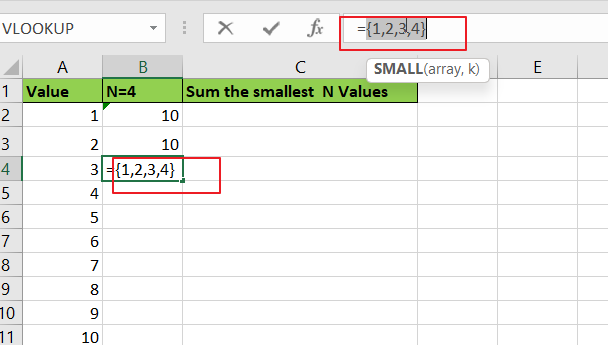Obviously, the final result is 1+2+3+4=10. Select SUMPRODUCT({1,2,3,4}) in the formula bar, press F9, 10 is displayed in formula bar.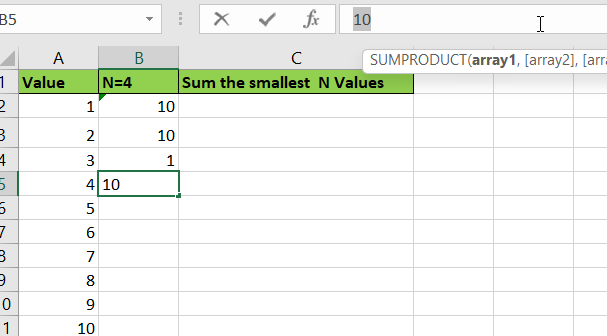1. In this instance, there are only 10 numbers in the range, and we only want to sum the smallest 4 number. If there are a large amount of numbers, and sum the smallest 100 or more numbers in the range, do we need to enter {1,2,3,4,…,100} in SMALL function? Obviously, it is very complex and troublesome. So, we can solve this problem by the help of ROW and INDIRECT

For example, if we want to sum the smallest 9 numbers in the range, we can enter =SUMPRODUCT(SMALL(A2:A11,ROW(INDIRECT(“1:9”)))) in B2. Then we can get correct value 45.In this case, INDIRECT function can return a valid reference based on entered texts. With the help of ROW, ROW(INDIRECT(“1:9”)) can convert “1:9” to {1,2,3,4,5,6,7,8,9}.2. If N number is saved in another cell, we can concentrate inside INDIRECT function. Enter =SUMPRODUCT(SMALL(A2:A11,ROW(INDIRECT(“1:”&B2)))) in C2 this time. See example below:In this case, when changing the number in B2, result in C2 will be automatically updated. We don’t need to adjust the formula or cell reference.3. In this case we can also use SUM function to replace SUMPRODUCT function. But SUMPRODUCT function can handle arrays directly without entering Ctrl + Shift + Enter, so we often apply SUMPRODUCT function in similar situations.

## SUMMARY

1.  SUMPRODUCT function can handle multiple arrays, and it sum up the products. And allows user defined range, wildcards, logical operators, expressions.
2. SMALL function can return the Nth smallest value in a range.
3. ROW(INDIRECT(“1:N”)) can return a set of numeric numbers in an array.
4. If N is in another cell, use “&” to concentrate them inside INDIRECT, for example ROW(INDIRECT(“1:”&B2)).

## Related Functions

• Excel SUMPRODUCT function
The Excel SUMPRODUCT function multiplies corresponding components in the given one or more arrays or ranges, and returns the sum of those products.The syntax of the SUMPRODUCT function is as below:= SUMPRODUCT (array1,[array2],…)…
• Excel INDIRECT function
The Excel INDIRECT function returns the cell reference based on a text string, such as: type the text string “A2” in B1 cell, it just a text string, so you can use INDIRECT function to convert text string as cell reference….
• Excel ROW function
The Excel ROW function returns the row number of a cell reference.The ROW function is a build-in function in Microsoft Excel and it is categorized as a Lookup and Reference Function.The syntax of the ROW function is as below:= ROW ([reference])….
• Excel SMALL function
The Excel SMALL function returns the smallest numeric value from the numbers that you provided. Or returns the smallest value in the array.The syntax of the SMALL function is as below:=SMALL(array,nth) …
• Excel SUM function
The Excel SUM function will adds all numbers in a range of cells and returns the sum of these values. You can add individual values, cell references or ranges in excel.The syntax of the SUM function is as below:= SUM(number1,[number2],…)

Sidebar